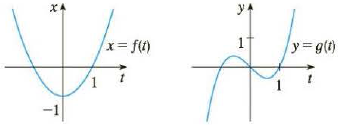Chapter 10, Problem 6RE

Chapter
Section
Textbook Problem

# Use the graphs of x = f(t) and y = g(t) to sketch the parametric curve x = f(t), y = g(t). Indicate with arrows the direction in which the curve is traced as t increases.To determine

To find: The parametric curve figure from the given equations.

Explanation

Given:

Equations are x=f(t) and y=g(t)

Calculation:

Consider the equation x=f(t) .

The figure shows the curve for the equation x=f(t) as shown in the figure (1).

From the above figure shows parabola curve where the variable t is in the limit of ±1

Therefore,

f(t)=k(t+1)(t1)

f(t)=k(t21) (1)

From the figure, f(0)=1

Substitute 0 for t in the equation (1).

f(t)=k(t21)f(0)=k(021)

f(0)=k(1) (2)

Calculate the value of k.

Substitute 1 for f(0) in the equation (2).

f(0)=k(1)1=k(1)k=1

Substitute 1 for k in the equation (1).

f(t)=k(t21)f(t)=1(t21)f(t)=t21

Assume t21=x . Therefore,

t21=xt=±x+1 (3)

Consider the equation y=g(t) .

From the above figure shows the polynomial curve where the variable t is 0,±1

Therefore, the equation is,

g(t)=k(t1)t(t+1) (4)

g(t)=kt(t21) (5)

From the graph, take t as 0

### Still sussing out bartleby?

Check out a sample textbook solution.

See a sample solution

#### The Solution to Your Study Problems

Bartleby provides explanations to thousands of textbook problems written by our experts, many with advanced degrees!

Get Started

#### In Problems 19-44, factor completely. 34.

Mathematical Applications for the Management, Life, and Social Sciences

#### True or False:

Study Guide for Stewart's Multivariable Calculus, 8th

#### sec1(23)= a) 3 b) 3 c) 6 d) 6

Study Guide for Stewart's Single Variable Calculus: Early Transcendentals, 8th

#### Define fraud and explain the safeguards that exist to prevent it.

Research Methods for the Behavioral Sciences (MindTap Course List)